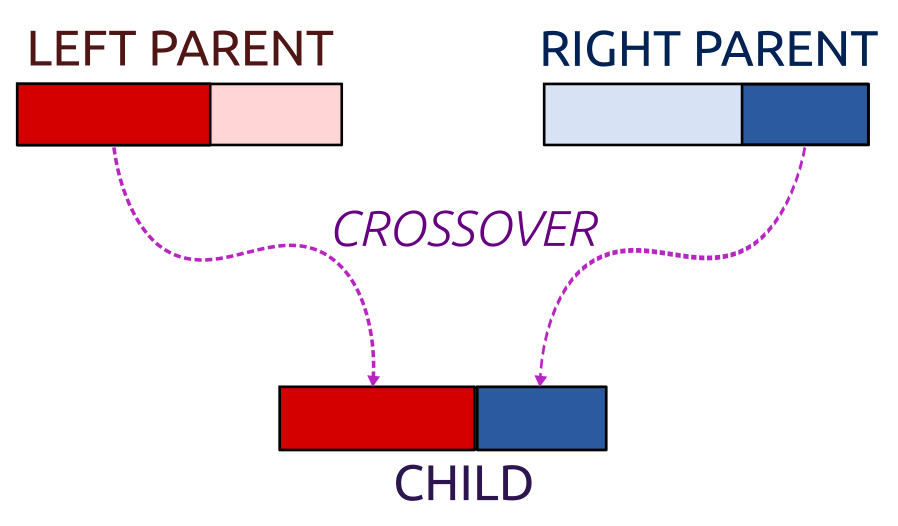## 8.3 Genetic Algorithms

### 8.3.1 Introduction

What if our optimisation problem cannot be solved reliably with gradient-based methods like those in optim() and we don’t have any custom solver for the task at hand?

There are a couple of useful metaheuristics in the literature that can serve this purpose.

Most of them rely on clever randomised search.

They are slow to run and don’t guarantee anything, but yet they still might be useful – better a solution than no solution at all.

There is a wide class of nature-inspired algorithms (that traditionally belong to the subfield of AI called computational intelligence or soft computing); see, e.g, (Simon 2013):

• evolutionary algorithms – inspired by the principle of natural selection

maintain a population of candidate solutions, let the “fittest” combine with each other to generate new “offspring” solutions.

• swarm algorithms

maintain a herd of candidate solutions, allow them to “explore” the environment, “communicate” with each other in order to seek the best spot to “go to”.

For example:

• ant colony
• bees
• cuckoo search
• particle sward
• krill herd
• other metaheuristics:

• harmony search
• memetic algorithm
• firefly algorithm

All of these sound fancy, but the general ideas behind them are pretty simple.

### 8.3.2 Overview of the Method

Genetic algorithms (GAs) are amongst the most popular evolutionary approaches.

They are based on Charles Darwin’s work on evolution by natural selection.

See (Goldberg, 1989) for a comprehensive overview and (Simon, 2013) for extensions.

Here is the general idea of a GA (there might be many) to minimise a given objective/fitness function $$f$$ over a given domain $$D$$.

1. Generate a random initial population of individuals – $$n_\text{pop}$$ points in $$D$$, e.g., $$n_\text{pop}=128$$

2. Repeat until some convergence criterion is not met:

1. evaluate the fitness of each individual
2. select the pairs of the individuals for reproduction, the fitter should be selected more eagerly
3. apply crossover operations to create offspring
4. slightly mutate randomly selected individuals
5. replace the old population with the new one

### 8.3.3 Example Implementation - GA for K-means

Initial setup:

set.seed(123)

# simulation parameters:
npop <- 32
niter <- 100

# randomly generate an initial population of size npop:
pop <- lapply(1:npop, function(i) X[sample(nrow(X), K),])

# evaluate fitness of each individual:
cur_fitness <- sapply(pop, get_fitness, X)
cur_best_fitness <- min(cur_fitness)
best_fitness <- cur_best_fitness

Each individual in the population is just the set of $$K$$ candidate cluster centres represented as a matrix in $$\mathbb{R}^{K\times p}$$.

Let’s assume that the fitness of each individual should be a function of the rank of the objective function’s value (smallest objective == highest rank == best fit).

For the crossover, we will sample pairs of individuals with probabilities proportional to their fitness.

selection <- function(cur_fitness) {
npop <- length(cur_fitness)
probs <- rank(-cur_fitness)
probs <- probs/sum(probs)
left  <- sample(npop, npop, replace=TRUE, prob=probs)
right <- sample(npop, npop, replace=TRUE, prob=probs)
cbind(left, right)
}

An example crossover combines each cluster centre in such a way that we take a few coordinates of the “left” parent and the remaining ones from the “right” parent (see below for an illustration):

crossover <- function(pop, pairs, K, p) {
old_pop <- pop
pop <- pop[pairs[,2]]
for (j in 1:length(pop)) {
wh <- sample(p-1, K, replace=TRUE)
for (l in 1:K)
pop[[j]][l,1:wh[l]] <-
old_pop[[pairs[j,1]]][l,1:wh[l]]
}
pop
}Mutation (occurring with a very small probability) substitutes some cluster centre with a random vector from the input dataset.

mutate <- function(pop, X, K) {
for (j in 1:length(pop)) {
if (runif(1) < 0.025) {
szw <- sample(1:K, 1)
pop[[j]][szw,] <- X[sample(nrow(X), length(szw)),]
}
}
pop
}

We also need a function that checks if the new cluster centres aren’t too far away from the input points.

If it happens that we have empty clusters, our solution is degenerate and we must correct it.

All “bad” cluster centres will be substituted with randomly chosen points from $$\mathbf{X}$$.

Moreover, we will recompute the cluster centres as the componentwise arithmetic mean of the closest points, just like in Lloyd’s algorithm, to speed up convergence.

recompute_mus <- function(pop, X, K) {
for (j in 1:length(pop)) {
# get nearest cluster centres for each point:
memb <- get.knnx(pop[[j]], X, 1)$nn.index sz <- tabulate(memb, K) # number of points in each cluster # if there are empty clusters, fix them: szw <- which(sz==0) if (length(szw)>0) { # random points in X will be new cluster centres pop[[j]][szw,] <- X[sample(nrow(X), length(szw)),] memb <- FNN::get.knnx(pop[[j]], X, 1)$nn.index
sz <- tabulate(memb, K)
}
# recompute cluster centres - componentwise average:
pop[[j]][,] <- 0
for (l in 1:nrow(X))
pop[[j]][memb[l],] <- pop[[j]][memb[l],]+X[l,]
pop[[j]] <- pop[[j]]/sz
}
pop
}

We are ready to build our genetic algorithm to solve the K-means clustering problem:

for (i in 1:niter) {
pairs <- selection(cur_fitness)
pop <- crossover(pop, pairs, K, p)
pop <- mutate(pop, X, K)
pop <- recompute_mus(pop, X, K)
# re-evaluate fitness:
cur_fitness <- sapply(pop, get_fitness, X)
cur_best_fitness <- min(cur_fitness)
# give feedback on what's going on:
if (cur_best_fitness < best_fitness) {
best_fitness <- cur_best_fitness
best_mu <- pop[[which.min(cur_fitness)]]
cat(sprintf("%5d: f_best=%10.5f\n", i, best_fitness))
}
}

##     1: f_best=435638.52165
##     2: f_best=428808.89706
##     4: f_best=428438.45125
##     6: f_best=422277.99136
##     8: f_best=421889.46265
print(get_fitness(best_mu, X))
##  421889.5
print(get_fitness(res_tried_very_hard, X))
##  421889.5

It works! :)